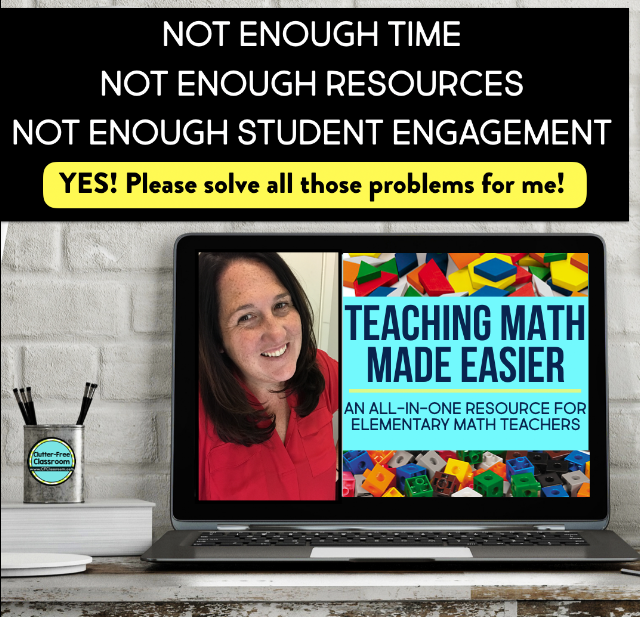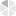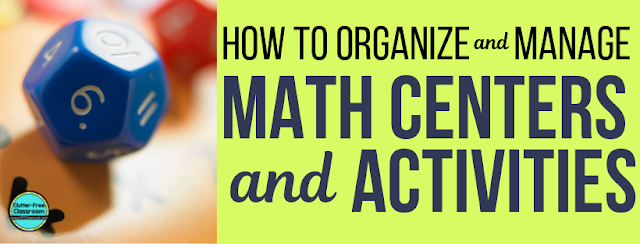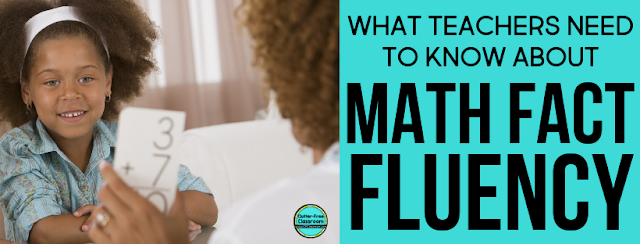# Math Fact Fluency

There are so many common misconceptions about math fact fluency. It's an incredibly important piece of students' elementary math education - as it is one of the biggest indicators to a child's future success in math classes. Read below to clear up any misconceptions you may have and strengthen your math fact fluency understanding and instruction.

This blog post will...
• define math fact fluency and explain how it is different than automaticity
• explain why it is important
• identify common misconceptions surrounding it and provide the information you need to successfully build fluent mathematicians in your classroomWHAT IS MATH FACT FLUENCY?
Math fact fluency is the ability to quickly recall addition, subtraction, multiplication, and division math facts through conceptual learning, fact strategies, and memorization. The four key components to determine mastery are 1) flexibility, 2) appropriate strategy use, efficiency, and accuracy. When students achieve automaticity of their facts, a common benchmark is that they can recall them in two seconds or less (30 problems in 60 seconds). This skill is essential for students to master and tremendously impacts their future in mathematics; however, it is important to note that students naturally progress at their own rate. As teachers, we need to provide a strong foundation for students (conceptual learning and strategies) followed by daily opportunities to practice (memorization) in fun and engaging ways.FLUENCY VS. AUTOMATICITY
Fluency and automaticity are often used interchangeably; however, they are not synonyms. Fluency is the ability to flexibly apply strategies in an efficient and accurate way. Automaticity, on the other hand, is being able to provide an automatic response. When a student is fluent, they are automatic. When a student is automatic, they are not necessarily fluent.3 REASONS WHY MATH FACT FLUENCY IS IMPORTANT

1. Contributes to the ability to solve more difficult problems quicker and more accurately.
2. Leads to success in their future mathematics courses and careers.
3. Builds confidence in math ability.WHAT ARE COMMON MISCONCEPTIONS ABOUT MATH FACT FLUENCY?

1. The best way to assess math fact fluency is through timed tests (e.g. mad minutes).
• Timed tests give limited information about what strategies the student used.
• They also do not give information on how flexibly the student used their strategies.
• It sends the wrong message to your students. Fast is not always best. It is important to celebrate strategies rather than speed.
• Research shows that timed tests can actually have a negative impact on students and their progress towards being fluent in math facts.
• Try a math running record or math interview!

1. The best way to improve math fact fluency is through drilling facts.
• Never use fact drills unless the student has developed a strong understanding of the concept and efficient strategies for the facts being practiced.
• Games are a very effective tools to helping students master facts in a fun and engaging way.

1. Math fact fluency is all about memorizing facts.
• Math fact fluency is about understanding concepts and applying strategies. Automaticity will come after a student masters those two pieces.WHAT IS THE SCOPE AND SEQUENCE OF TEACHING MATH FACT FLUENCY?

1. Conceptual Learning
• Teach the meaning of the operations so students truly understand them.
• Use and give students the opportunity to use lots of manipulatives and models.

1. Fact Strategies
• Explicitly teach strategies (e.g. counting on and doubles).
• Provide lots of opportunities to practice and apply these strategies.
• Emphasize relationships and connections between operations.
• Use and give students the opportunity to use lots of manipulatives and models.
• Require students to explain what strategies they used and justify their response.

1. Memorization of Basic Facts
• Provide varied and daily opportunities to practice.
• Students should be able to recall a fact within 2 seconds.
• Practice facts using games. Keep it fun!
• Differentiate practice.WHAT DOES THE PROGRESSION OF MATH FACT FLUENCY LOOK LIKE ACROSS GRADE LEVELS?

Kindergarten
• Fluently add and subtract within 5.

• Add and subtract within 20, demonstrating fluency for addition and subtraction within 10. Use strategies such as counting on; making ten (e.g., 8 + 6 = 8 + 2 + 4 = 10 + 4 = 14); decomposing a number leading to a ten (e.g., 13 - 4 = 13 - 3 - 1 = 10 - 1 = 9); using the relationship between addition and subtraction (e.g., knowing that 8 + 4 = 12, one knows 12 - 8 = 4); and creating equivalent but easier or known sums (e.g., adding 6 + 7 by creating the known equivalent 6 + 6 + 1 = 12 + 1 = 13).

• Fluently add and subtract within 20 using mental strategies.2 By end of Grade 2, know from memory all sums of two one-digit numbers.

• Fluently add and subtract within 1000 using strategies and algorithms based on place value, properties of operations, and/or the relationship between addition and subtraction.
• Fluently multiply and divide within 100, using strategies such as the relationship between multiplication and division (e.g., knowing that 8 × 5 = 40, one knows 40 ÷ 5 = 8) or properties of operations. By the end of Grade 3, know from memory all products of two one-digit numbers.

• Fluently add and subtract multi-digit whole numbers using the standard algorithm.

• Fluently multiply multi-digit whole numbers using the standard algorithm.WHAT ARE WAYS I CAN HELP MY STUDENTS PRACTICE THEIR MATH FACTS?
• Send home information and resources to families about math fact fluency.
• Send home games for students to play with their families for homework.
• Play math games in class.
• Commit to daily practice.
• Listen to and sing songs.
• Use a math app.
• Utilize a website-based game.
• Track student progress in your notes and on a bulletin board.
• Invite students to track their own progress.
• Play Xtra Math.
• Celebrate strategies and successes.
• Model and instill a growth-mindset and positive attitude toward math facts.HOW DO I ASSESS MATH FACT FLUENCY?
When getting the full picture of a students mastery of math facts, it is important to consider four components: 1) Flexibility, 2) appropriate strategy use, 3) efficiency, and 4) accuracy.  Data should also include which facts students know from memory.  Below is a list of tools commonly used to assess math fact fluency.

• Math Running Records (Affiliate Link to Dr. Nikki Newton’s book)
• Math Interviews
• Observation during games
• Observations during whole group discussion
• Oral or written explanations of what strategy was chosen and why
• Reflections in math journal### READ ALL THE BLOG POSTS IN THE ELEMENTARY MATH SERIES:By the way, if you are not already subscribed to The Clutter-Free Classroom newsletter I encourage you sign up. Subscribers receive weekly tips for organizing and managing a classroom as well as exclusive free printables. You can sign up here.